Let me take another stroll down memory lane, this time recalling not my first kiss but my first encounter with multiplication. They showed me these obscure hieroglyph thingies:

3*2 = 6

3*3 = 9

3*4 = 12

etc.

Then they wanted me to memorize them. As. If. I wish I could say that my math skills prospered despite my inability to multiply two by two to save my life. On the other hand, I must've been saved from my innumeracy somehow, or I wouldn't be writing this, now would I? Was it a devoted teacher with an especially persuasive ruler? Proper parenting? God? None of the above. It was this nifty trick I discovered:

3*2 = 3 + 3

3*3 = 3 + 3 + 3

3*4 = 3 + 3 + 3 + 3

etc.

So, multiplication is just a shorthand for taking a number and adding it to itself. Rinse, repeat. Armed with this knowledge, I could approach the hairiest calculus problems undaunted. And come to think of it, haven't we just dealt with such a problem? Here's a summation of my early kissing career, as described above:

0.1 + 0.1 + 0.1 + 0.1 + 0.1 + 0.1 = 0.4

All we did was take the BNumber 0.1 and add it to itself five times, resulting in six times 0.1. Of course, it would have been much simpler to use multiplication for this purpose. In the BNumber world, this would be achieved by the following operation:

0.1 * 0.85 = 0.4

Looks ridiculous, doesn't it? You were willing to indulge me that bit about BSum, but this is really too much, isn't it? Well, again I ask for your patience - there really is a method to this madness. If you keep an open mind I just might be able to bring you around to the thinking of Storytron's in-house eggheads.

Let's begin by considering some Deep Truths about multiplication. Multiplication always starts with a value X (let's assume it's a positive number). You then take another such X and add it to the first:

X + X = 2X

You then take the result and you add another X to it. You keep doing this for a specified number of times (Y times). This gives you a number - the product of X and Y. In other words:

X*Y = YX.

Rocket science, right? Now, there are a couple of kinks in this system, depending on the value of Y. First of all, if Y is zero we don't perform this process even once. We do exactly nothing, and that's why we always end up with zero. If Y is 1, we add a first X, but don't add anything else, and that's why we always end up with X. Let's draw a schematic map of multiplication, using zero and 1 as our landmarks: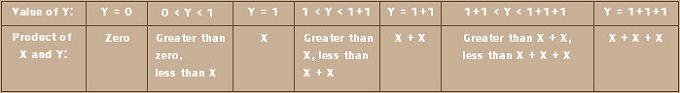Big deal, huh? Ten times zero is zero. Ten times 0.5 is 5. Ten times 1 is 10. Ten times 1.5 is 15. Ten times 2 is 20. So on. Doesn't take an Einstein. But there is one extremely important point here: this whole system is built around multiples of the number 1. Let's take that concept for a whirl. Suppose my alien abductors from the BSum section wanted to learn about the meaning of 1 for multiplication. How would I define it for them?

· When you multiply X by Y multiples of 1, you get Y multiples of X.
· When you multiply X by a value that is greater than Y multiples of 1, but less than Y+1 multiples of 1, you get a number which is greater than Y multiples of X, but less than Y+1 multiples of X.

So far for normal numbers. What about BNumbers? Well, there is indeed a BNumber that fits the above definition: 0.5. In the BNumber world:

· When you multiply X by Y multiples of 0.5, you get Y multiples of X.
· When you multiply X by a value that is greater than Y multiples of 0.5, but less then Y+1 multiples of 0.5, you get a number which is greater than Y multiples of X, but less than Y+1 multiples of X.

So, while normal multiplication works in multiples of 1, BNumber multiplication works in multiples of 0.5. What are the multiples of 0.5? Remember that 0.5 is a BNumber, so to calculate its multiples we must use BSum. Once we've done that, we can draw a map of BNumber multiplication.

Let's imagine a 0.5k kiss - a much more potent kiss than the kind we discussed above. Those were the tentative pecks of infatuated teenagers, while this is an all-in, true-love kind of kiss. You can only have it after you've had some experience, so let's start out my kissitude at 0.5. How would another five such kisses affect my kissitude? Have a look: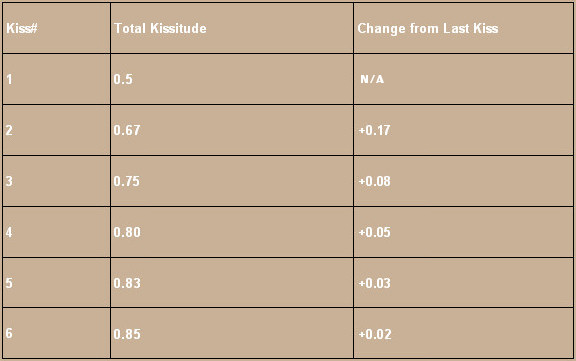So the first five multiples of 0.5 are 0.67, 0.75, 0.80, 0.83 and 0.85. Therefore, in the BNumber world, multiplication looks like this:

X * 0.5 = X

X * 0.67 = X+X

X * 0.75 = X+X+X

X * 0.80 = X+X+X+X

X * 0.83 = X+X+X+X+X

X * 0.85 = X+X+X+X+X+X

Now we can draw a schematic map of BNumber multiplication: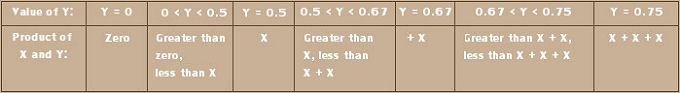Now let's go back to our original, 0.1k kisses. But this time, let's use BProduct instead of BSum to describe how they affected my kissitude. To calculate the effect of one single kiss, we would multiply 0.1 by a single multiple of 0.5:

0.1 * 0.5 = 0.1

To calculate the effect of two kisses, we would again multiply 0.1, this time by two multiples of 0.5:

0.1 * (0.5 + 0.5) =
0.1 * 0.67 = 0.18

To make three kisses, we would multiply 0.1 by three multiples of 0.5:

0.1 * (0.5 + 0.5 + 0.5) =
0.1 * 0.75 = 0.25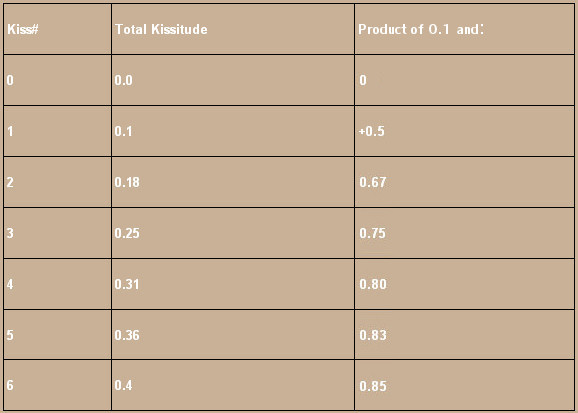Bounded Division

Suppose my alien abductors wanted to erase half my kisses from my memory. Their starting point would be six kisses:

0.1 + 0.1 + 0.1 + 0.1 + 0.1 + 0.1 = 0.4

Their desired endpoint would be three kisses:

0.1 + 0.1 + 0.1 = 0.25

So, how do you turn 0.4 into 0.25? Well, let's start with what we know; how to turn 0.25 into 0.4:

0.25 + 0.1 + 0.1 + 0.1 = 0.4

We can also write this thusly:

0.25 + 0.25 = 0.4

Now, 0.25 + 0.25 is just another way of saying 0.25 times two. And we already know how to do the BNumber equivalent of that: 0.25 * 0.67. It follows that:

0.25 * 0.67 = 0.4

Now, division is just multiplication in reverse. So, if the product of 0.25 and 0.67 is 0.4, it follows that the quotient of 0.4 and 0.67 is 0.25. So:

0.4 / 0.67 = 0.25

This inversion works the same way for all multiples of 0.5. For example, suppose my abductors wanted to wipe out two thirds of my kissing memories. Their starting point would again be six kisses:

0.1 + 0.1 + 0.1 + 0.1 + 0.1 + 0.1 = 0.4

This time, their desired endpoint would be:

0.1 + 0.1 = 0.18

So let's start with what we know; how to turn 0.18 into 0.4:

0.18 + 0.1 + 0.1 + 0.1 + 0.1 = 0.4

Thus:

0.18 + 0.18 + 0.18 = 0.4

This is 0.18 times three, which in BNumbers makes:

0.18 * 0.75 = 0.4

Therefore:

0.4 / 0.75 = 0.18

UNumbers

A BNumber can be anything between -1 and 1, but sometimes you require a value between 0 and 1. The Operator BNumber2UNumber takes a BNumber and re-expresses it as a Unary Number, which is basically a BNumber except it can only be anything between 0 and 1. The following table shows examples of BNumbers and their corresponding UNumbers: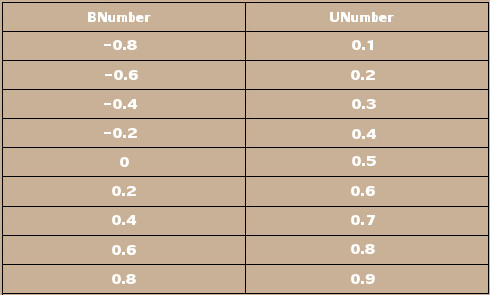The Operator UNumber2BNumber does the exact opposite, taking a UNumber and turning it into its corresponding BNumber.

Previous:  BNumbers and UNumbers
Bounded Multiplication